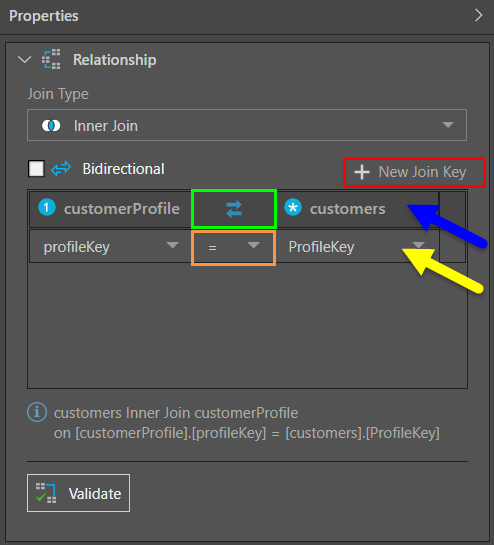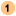# Join Keys

Database tables usually contain one or more key columns. Key columns generally list unique identifiers in order to uniquely identify rows in the database tables. Joins combine rows from multiple table based on related or common columns shared by them; these columns are usually key columns. The value (generally key columns) used to combine rows between tables is called a join key.

Using heuristics, Pyramid identifies the Key column(s) in each table, and creates table joins based on related or common key columns. Your model may contain tables that have more than one key column. In this case, you can edit the join key or add multiple join keys when editing a given join.

## Editing Join Keys

Join keys are edited from the Relationship window in the Properties panel of the selected join. The join key table shows the tables being joined and the join direction (blue arrow below), the join key columns (yellow arrow), and the join key operator (orange highlight):### Add or Edit Join Keys

To add a new join key, click 'New Join Key' (red highlight above), and then select the required columns and operator. Join key columns can be changed simply by selecting a different column from the drop-down list.

#### Multi-Join Keys

You may have some tables containing multiple identical key columns. In this case, you can edit the join to include multiple join keys. In this way, you can join 2 tables by multiple shared key columns, via a single join. The join type and direction for each join key will be the same, but the join key operation can be different.

### Join Direction

Click the double arrows icon to change the direction of the join (green highlight above). The table denoted by theicon is the table on the 'left' (the table from which the join originates, known as the primary table). The table denoted by theicon is the table on the 'right' (the table to which the join is connected, known as the foreign table). The primary table is the table containing the primary key column, which the foreign table contains the foreign key column.

Typically, joins will be one-way (unidirectional), but there may be circumstances in which your want the data between 2 tables to flow in both directions (bidirectional joins). this can be achieved by enabling the 'Bidirectional' option.

### Join Key Operators

Select the join operator from the drop-down list (purple highlight below). By default the join operation is set to = but it can be changed to a non equal operator if required. Equal joins are used to join rows where the primary key columns in each table match. Non equal functions are used to create joins where the key values don't match.

Non equal joins are used for a range of purposes, like checking for duplicate data, generating running totals, or to join according to a range of values or dates. You might want to find the names of salespeople who were hired less than 3 months ago, so their on-boarding and progress can be tracked. Or you might want to find a list of students who are not studying Physics this semester, so you can check if whether or not they have enough credits.

The join operators available are:

• = joins rows where the key in both tables is the same.
• < > joins rows where the key in both tables is not the same.
• > joins rows where the key in the left table is greater than the key in the right table.
• > = joins rows where the key in the left table is greater than or equal to the key in the right table.
• < joins rows where the key in the right table is smaller than the key in the right table.
• < = joins rows where the key in the right table is smaller than or equal to the key in the right table.